### Day 65 - Integration using the Natural Logarithmic Function - 11.19.15

 Update N/A ChallengePosted on the boardReview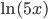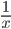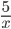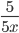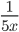none of the aboveDifferentiate.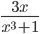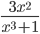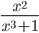none of the aboveDifferentiate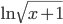.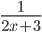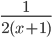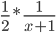both b and cnone of the aboveDifferentiate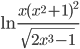.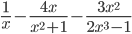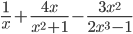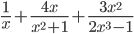none of the above LessonIntegration using the Natural Logarithmic Function (checkpoints) Posted on the board at the end of the blockHomeworkWNQ the following: Help Request List Standard(s) APC.9Apply formulas to find derivatives.Includes:derivatives of algebraic, trigonometric, exponential, logarithmic, and inverse trigonometric functionsderivations of sums, products, quotients, inverses, and composites (chain rule) of elementary functionsderivatives of implicitly defined functionshigher order derivatives of algebraic, trigonometric, exponential, and logarithmic, functions